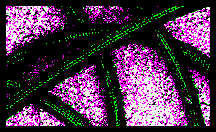# The Physics Department- Mechanics, Curved MotionObviously, curved motion is motion along a path that is not straight. Therefore, an object following any type of curved motion will necessarily be changing its direction as it moves. This change in direction constitutes a change in velocity, since velocity is a vector, and, thus, velocity has both a size, the speed, and a direction. So, if an object travels in a curved path, it changes velocity, and, thus, accelerates. This acceleration must be tied to a force. Newton's laws of motion explain this. Therefore, whenever an object travels in a curved path, there must be an unbalanced force acting upon it.

It is important to understand that all this may occur without a change in speed. An automobile being driven in a large circle at a constant speed of 30 mph will be said to be accelerating, and there must be an unbalanced force applied to it.

Custom Search